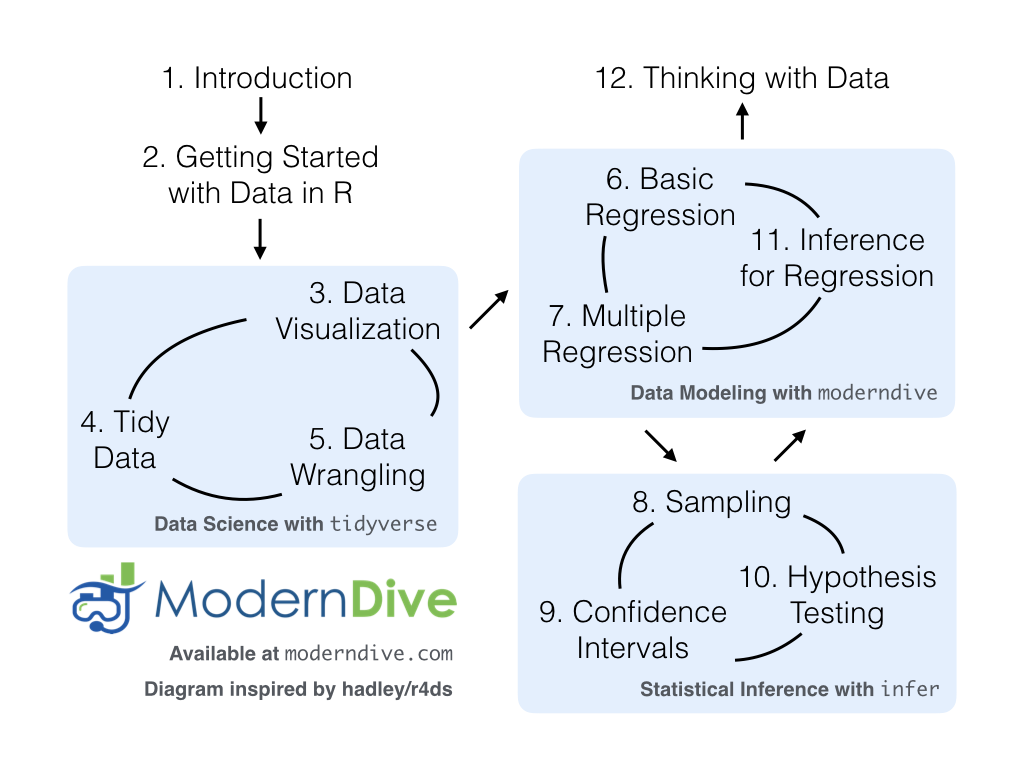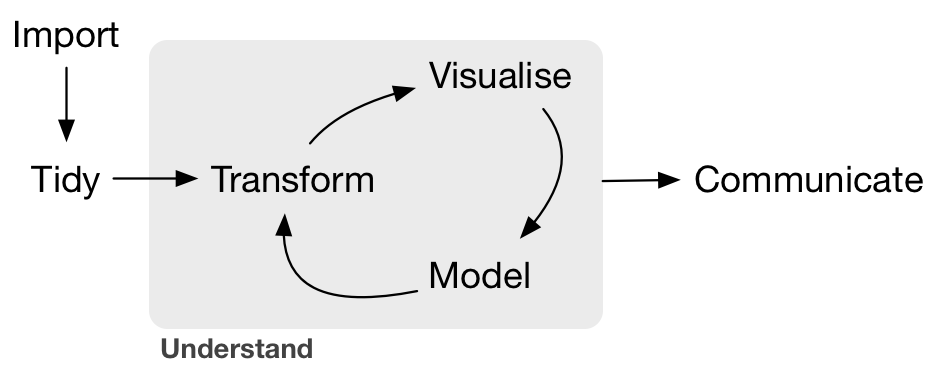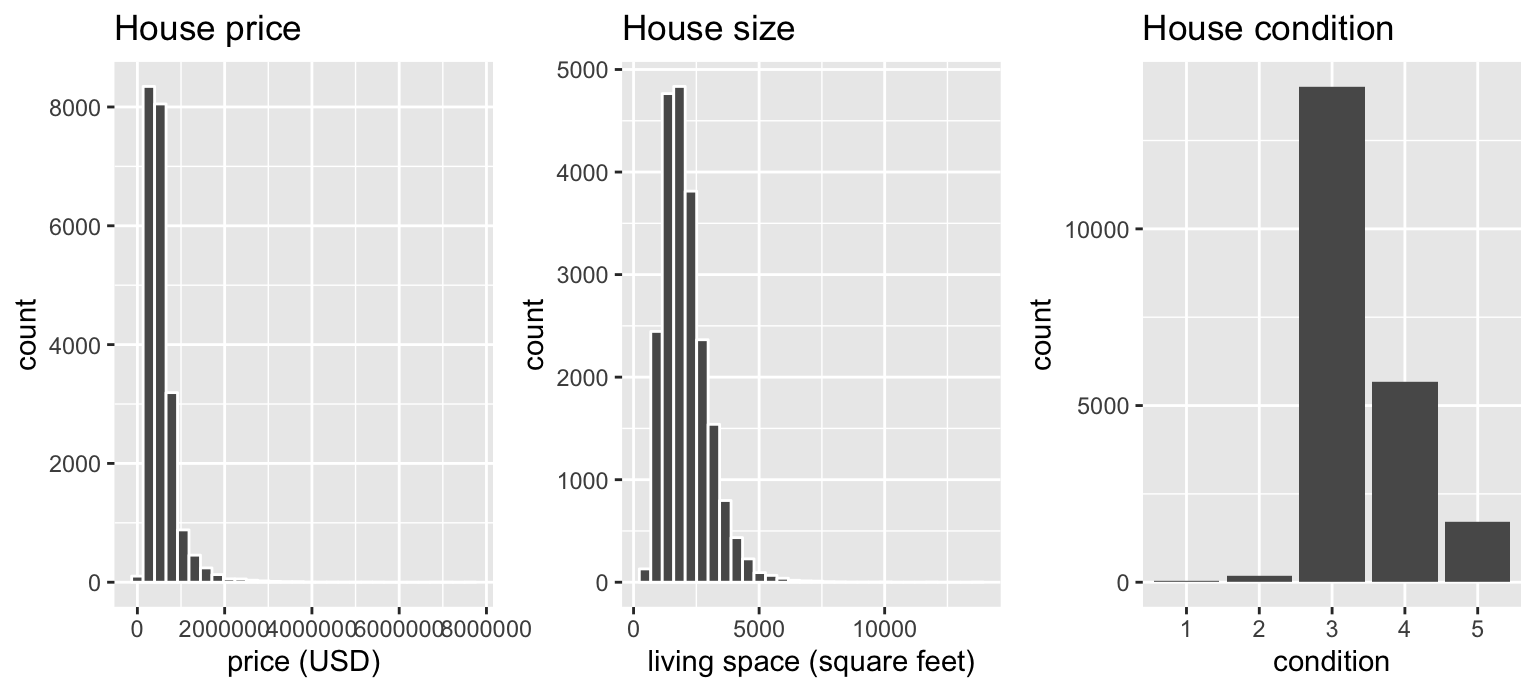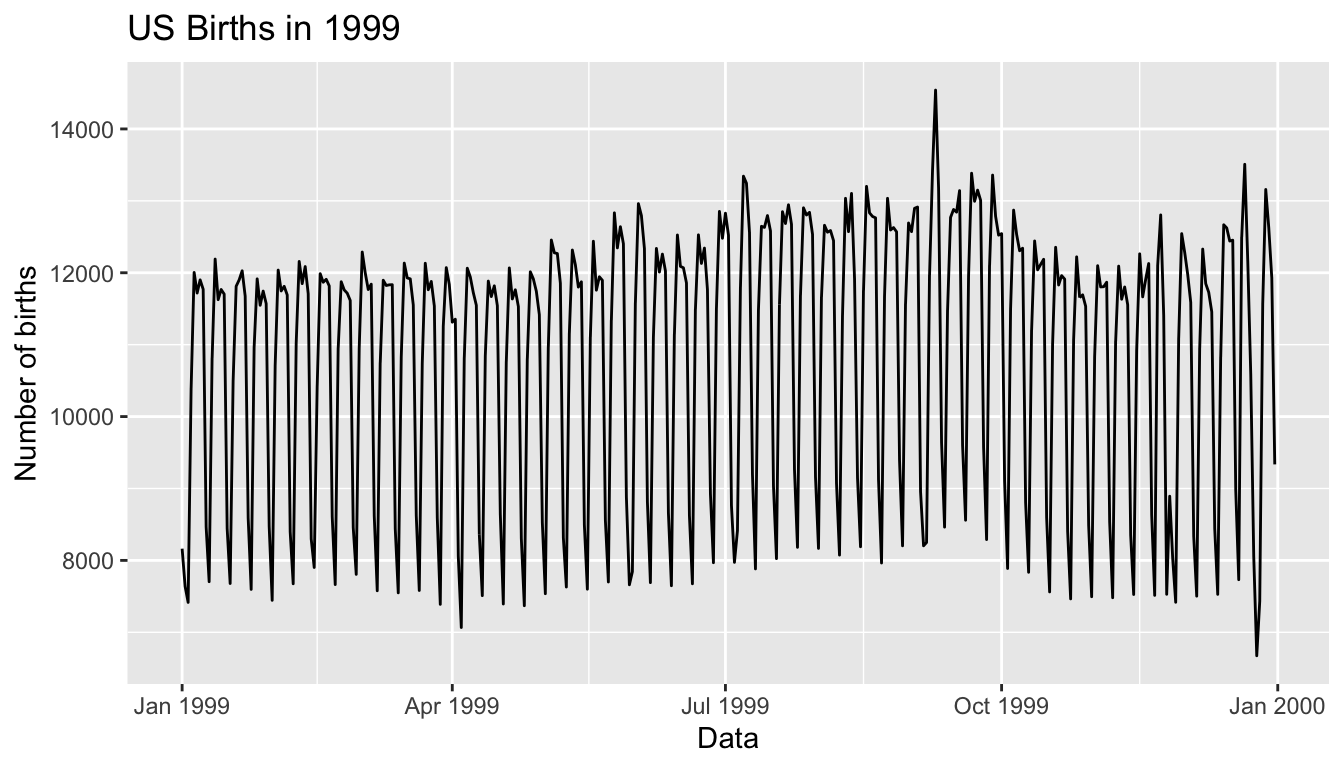Chapter 12 Thinking with Data

In preparation for our first print edition to be published by CRC Press in Fall 2019, we’re remodeling this chapter a bit. Don’t expect major changes in content, but rather only minor changes in presentation. Our remodeling will be complete and available online at ModernDive.com by early Summer 2019!

Recall in Section 1.1 “Introduction for students” and at the end of chapters throughout this book, we displayed the “ModernDive flowchart” mapping your journey through this book.FIGURE 12.1: ModernDive Flowchart

Let’s get a refresher of what you’ve covered so far. You first got started with with data in Chapter 2, where you learned about the difference between R and RStudio, started coding in R, started understanding what R packages are, and explored your first dataset: all domestic departure flights from a New York City airport in 2013. Then:

1. Data science: You assembled your data science toolbox using tidyverse packages. In particular:
• Ch.3: Visualizing data via the ggplot2 package.
• Ch.5: Understanding the concept of “tidy” data as a standardized data input format for all packages in the tidyverse
• Ch.4: Wrangling data via the dplyr package.
2. Data modeling: Using these data science tools and helper functions from the moderndive package, you started performing data modeling. In particular:
• Ch.6: Constructing basic regression models.
• Ch.7: Constructing multiple regression models.
3. Statistical inference: Once again using your newly acquired data science tools, we unpacked statistical inference using the infer package. In particular:
• Ch.8: Understanding the role that sampling variability plays in statistical inference using both tactile and virtual simulations of sampling from a “bowl” with an unknown proportion of red balls.
• Ch.9: Building confidence intervals.
• Ch.10: Conducting hypothesis tests.
4. Data modeling revisited: Armed with your new understanding of statistical inference, you revisited and reviewed the models you constructed in Ch.6 & Ch.7. In particular:
• Ch.11: Interpreting both the statistical and practice significance of the results of the models.

All this was our approach of guiding you through your first experiences of “thinking with data”, an expression originally coined by Diane Lambert of Google. How the philosophy underlying this expression guided our mapping of the flowchart above was well put in the introduction to the “Practical Data Science for Stats” collection of preprints focusing on the practical side of data science workflows and statistical analysis, curated by Jennifer Bryan and Hadley Wickham:

There are many aspects of day-to-day analytical work that are almost absent from the conventional statistics literature and curriculum. And yet these activities account for a considerable share of the time and effort of data analysts and applied statisticians. The goal of this collection is to increase the visibility and adoption of modern data analytical workflows. We aim to facilitate the transfer of tools and frameworks between industry and academia, between software engineering and statistics and computer science, and across different domains.

In other words, in order to be equipped to “think with data” in the 21st century, future analysts need preparation going through the entirety of the “Data/Science Pipeline” we also saw earlier and not just parts of it.FIGURE 12.2: Data/Science Pipeline

In Section 12.1, we’ll take you through full-pass of the “Data/Science Pipeline” where we’ll analyze the sale price of houses in Seattle, WA, USA. In Section 12.2, we’ll present you with examples of effective data storytelling, in particular the articles from the data journalism website FiveThirtyEight.com, many of whose source datasets are accessible from the fivethirtyeight R package.

Needed packages

Let’s load all the packages needed for this chapter (this assumes you’ve already installed them). Read Section 2.3 for information on how to install and load R packages.

library(ggplot2)
library(dplyr)
library(moderndive)
library(fivethirtyeight)

12.1 Case study: Seattle house prices

Kaggle.com is a machine learning and predictive modeling competition website that hosts datasets uploaded by companies, governmental organizations, and other individuals. One of their datasets is the House Sales in King County, USA consisting of homes sold in between May 2014 and May 2015 in King County, Washington State, USA, which includes the greater Seattle metropolitan area. This CC0: Public Domain licensed dataset is included in the moderndive package in the house_prices data frame, which we’ll refer to as the “Seattle house prices” dataset.

The dataset consists 21,613 houses and 21 variables describing these houses; for a full list of these variables see the help file by running ?house_prices in the console. In this case study, we’ll create a model using multiple regression where:

• The outcome variable $$y$$ is the sale price of houses
• The two explanatory/predictor variables we’ll use are :
1. $$x_1$$: house size sqft_living, as measured by square feet of living space, where 1 square foot is about 0.09 square meters.
2. $$x_2$$: house condition, a categorical variable with 5 levels where 1 indicates “poor” and 5 indicates “excellent.”

Let’s load all the packages needed for this case study (this assumes you’ve already installed them). If needed, read Section 2.3 for information on how to install and load R packages.

library(ggplot2)
library(dplyr)
library(moderndive)

12.1.1 Exploratory data analysis (EDA)

A crucial first step before any formal modeling is an exploratory data analysis, commonly abbreviated at EDA. Exploratory data analysis can give you a sense of your data, help identify issues with your data, bring to light any outliers, and help inform model construction. There are three basic approaches to EDA:

1. Most fundamentally, just looking at the raw data. For example using RStudio’s View() spreadsheet viewer or the glimpse() function from the dplyr package
2. Creating visualizations like the ones using ggplot2 from Chapter 3
3. Computing summary statistics using the dplyr data wrangling tools from Chapter 4

First, let’s look the raw data using View() and the glimpse() function. Explore the dataset. Which variables are numerical and which are categorical? For the categorical variables, what are their levels? Which do you think would useful variables to use in a model for house price? In this case study, we’ll only consider the variables price, sqft_living, and condition. An important thing to observe is that while the condition variable has values 1 through 5, these are saved in R as fct factors i.e. R’s way of saving categorical variables. So you should think of these as the “labels” 1 through 5 and not the numerical values 1 through 5.

View(house_prices)
glimpse(house_prices)
Observations: 21,613
Variables: 21
$id <chr> "7129300520", "6414100192", "5631500400", "2487200875",…$ date          <date> 2014-10-13, 2014-12-09, 2015-02-25, 2014-12-09, 2015-0…
$price <dbl> 221900, 538000, 180000, 604000, 510000, 1225000, 257500…$ bedrooms      <int> 3, 3, 2, 4, 3, 4, 3, 3, 3, 3, 3, 2, 3, 3, 5, 4, 3, 4, 2…
$bathrooms <dbl> 1.00, 2.25, 1.00, 3.00, 2.00, 4.50, 2.25, 1.50, 1.00, 2…$ sqft_living   <int> 1180, 2570, 770, 1960, 1680, 5420, 1715, 1060, 1780, 18…
$sqft_lot <int> 5650, 7242, 10000, 5000, 8080, 101930, 6819, 9711, 7470…$ floors        <dbl> 1.0, 2.0, 1.0, 1.0, 1.0, 1.0, 2.0, 1.0, 1.0, 2.0, 1.0, …
$waterfront <lgl> FALSE, FALSE, FALSE, FALSE, FALSE, FALSE, FALSE, FALSE,…$ view          <int> 0, 0, 0, 0, 0, 0, 0, 0, 0, 0, 0, 0, 0, 0, 0, 3, 0, 0, 0…
$condition <fct> 3, 3, 3, 5, 3, 3, 3, 3, 3, 3, 3, 4, 4, 4, 3, 3, 3, 4, 4…$ grade         <fct> 7, 7, 6, 7, 8, 11, 7, 7, 7, 7, 8, 7, 7, 7, 7, 9, 7, 7, …
$sqft_above <int> 1180, 2170, 770, 1050, 1680, 3890, 1715, 1060, 1050, 18…$ sqft_basement <int> 0, 400, 0, 910, 0, 1530, 0, 0, 730, 0, 1700, 300, 0, 0,…
$yr_built <int> 1955, 1951, 1933, 1965, 1987, 2001, 1995, 1963, 1960, 2…$ yr_renovated  <int> 0, 1991, 0, 0, 0, 0, 0, 0, 0, 0, 0, 0, 0, 0, 0, 0, 0, 0…
$zipcode <fct> 98178, 98125, 98028, 98136, 98074, 98053, 98003, 98198,…$ lat           <dbl> 47.5, 47.7, 47.7, 47.5, 47.6, 47.7, 47.3, 47.4, 47.5, 4…
$long <dbl> -122, -122, -122, -122, -122, -122, -122, -122, -122, -…$ sqft_living15 <int> 1340, 1690, 2720, 1360, 1800, 4760, 2238, 1650, 1780, 2…
$sqft_lot15 <int> 5650, 7639, 8062, 5000, 7503, 101930, 6819, 9711, 8113,… Let’s now perform the second possible approach to EDA: creating visualizations. Since price and sqft_living are numerical variables, an appropriate way to visualize of these variables’ distributions would be using a histogram using a geom_histogram() as seen in Section 3.5. However, since condition is categorical, a barplot using a geom_bar() yields an appropriate visualization of its distribution. Recall from Section 3.8 that since condition is not “pre-counted”, we use a geom_bar() and not a geom_col(). In Figure 12.3, we display all three of these visualizations at once. # Histogram of house price: ggplot(house_prices, aes(x = price)) + geom_histogram(color = "white") + labs(x = "price (USD)", title = "House price") # Histogram of sqft_living: ggplot(house_prices, aes(x = sqft_living)) + geom_histogram(color = "white") + labs(x = "living space (square feet)", title = "House size") # Barplot of condition: ggplot(house_prices, aes(x = condition)) + geom_bar() + labs(x = "condition", title = "House condition")FIGURE 12.3: Exploratory visualizations of Seattle house prices data We observe the following: 1. In the histogram for price: • Since e+06 means $$10^6$$, or one million, we see that a majority of houses are less than 2 million dollars. • The x-axis stretches out far to the right to 8 million dollars, even though there appear to be no houses. 2. In the histogram for size sqft_living • Most houses appear to have less than 5000 square feet of living space. For comparison a standard American football field is about 57,600 square feet, where as a standard soccer AKA association football field is about 64,000 square feet. • The x-axis exhibits the same stretched out behavior to the right as for price 3. Most houses are of condition 3, 4, or 5. In the case of price, why does the x-axis stretch so far to the right? It is because there are a very small number of houses with price closer to 8 million; these prices are outliers in this case. We say variable is “right skewed” as exhibited by the long right tail. This skew makes it difficult to compare prices of the less expensive houses as the more expensive houses dominate the scale of the x-axis. This is similarly the case for sqft_living. Let’s now perform the third possible approach to EDA: computing summary statistics. In particular, let’s compute 4 summary statistics using the summarize() data wrangling verb from Section 4.3. • Two measures of center: the mean and median • Two measures of variability/spread: the standard deviation and interquartile-range (IQR = 3rd quartile - 1st quartile) house_prices %>% summarize( mean_price = mean(price), median_price = median(price), sd_price = sd(price), IQR_price = IQR(price) ) # A tibble: 1 x 4 mean_price median_price sd_price IQR_price <dbl> <dbl> <dbl> <dbl> 1 540088. 450000 367127. 323050 Observe the following: 1. The mean price of$540,088 is larger than the median of $450,000. This is because the small number of very expensive outlier houses prices are inflating the average, whereas since the median is the “middle” value, it is not as sensitive to such large values at the high end. This is why the news typically reports median house prices and not average house prices when describing the real estate market. We say here that the median more “robust to outliers” than the mean. 2. Similarly, while both the standard deviation and IQR are both measures of spread and variability, the IQR is more “robust to outliers.” If you repeat the above summarize() for sqft_living, you’ll find a similar relationship between mean vs median and standard deviation vs IQR given its similar right-skewed nature. Is there anything we can do about this right-skew? Again, this could potentially be an issue because we’ll have a harder time discriminating between houses at the lower end of price and sqft_living, which might lead to a problem when modeling. We can in fact address this issue by using a log base 10 transformation, which we cover next. 12.1.2 log10 transformations At its simplest, log10() transformations returns base 10 logarithms. For example, since $$1000 = 10^3$$, log10(1000) returns 3. To undo a log10-transformation, we raise 10 to this value. For example, to undo the previous log10-transformation and return the original value of 1000, we raise 10 to this value $$10^{3}$$ by running 10^(3) = 1000. log-transformations allow us to focus on multiplicative changes instead of additive ones, thereby emphasizing changes in “orders of magnitude.” Let’s illustrate this idea in Table 12.1 with examples of prices of consumer goods in US dollars. TABLE 12.1: log10-transformed prices, orders of magnitude, and examples Price log10(Price) Order of magnitude Examples$1 0 Singles Cups of coffee
$10 1 Tens Books$100 2 Hundreds Mobile phones
$1,000 3 Thousands High definition TV’s$10,000 4 Tens of thousands Cars
$100,000 5 Hundreds of thousands Luxury cars & houses$1,000,000 6 Millions Luxury houses

Let’s break this down:

1. When purchasing a cup of coffee, we tend to think of prices ranging in single dollars e.g. $2 or$3. However when purchasing say mobile phones, we don’t tend to think in prices in single dollars e.g. $676 or$757, but tend to round to the nearest unit of hundreds of dollars e.g. $200 or$500.
2. Let’s say we want to know the log10-transformed value of $76. Even if this would be hard to compute without a calculator, we know that its log10 value is between 1 and 2, since$76 is between $10 and$100. In fact, log10(76) is 1.880814.
3. log10-transformations are monotonic, meaning they preserve orderings. So if Price A is lower than Price B, then log10(Price A) will also be lower than log10(Price B).
4. Most importantly, increments of one in log10 correspond to multiplicative changes and not additive ones. For example, increasing from log10(Price) of 3 to 4 corresponds to a multiplicative increase by a factor of 10: $100 to$1000.

Let’s create new log10-transformed versions of the right-skewed variable price and sqft_living using the mutate() function from Section 4.5, but we’ll give the latter the name log10_size, which is a little more succinct and descriptive a variable name.

house_prices <- house_prices %>%
mutate(
log10_price = log10(price),
log10_size = log10(sqft_living)
)

Let’s first display the before and after effects of this transformation on these variables for only the first 10 rows of house_prices:

house_prices %>%
select(price, log10_price, sqft_living, log10_size)
# A tibble: 10 x 4
price log10_price sqft_living log10_size
<dbl>       <dbl>       <int>      <dbl>
1  221900        5.35        1180       3.07
2  538000        5.73        2570       3.41
3  180000        5.26         770       2.89
4  604000        5.78        1960       3.29
5  510000        5.71        1680       3.23
6 1225000        6.09        5420       3.73
7  257500        5.41        1715       3.23
8  291850        5.47        1060       3.03
9  229500        5.36        1780       3.25
10  323000        5.51        1890       3.28

Observe in particular:

Learning check

(LC12.1) Repeat the regression modeling in Subsection 12.1.4 and the prediction making you just did on the house of condition 5 and size 1900 square feet in Subsection 12.1.5, but using the parallel slopes model you visualized in Figure 12.6. Hint: it’s $524,807! 12.2 Case study: Effective data storytelling Note: This section is still under construction. If you would like to contribute, please check us out on GitHub at https://github.com/moderndive/moderndive_book.As we’ve progressed throughout this book, you’ve seen how to work with data in a variety of ways. You’ve learned effective strategies for plotting data by understanding which types of plots work best for which combinations of variable types. You’ve summarized data in table form and calculated summary statistics for a variety of different variables. Further, you’ve seen the value of inference as a process to come to conclusions about a population by using a random sample. Lastly, you’ve explored how to use linear regression and the importance of checking the conditions required to make it a valid procedure. All throughout, you’ve learned many computational techniques and focused on reproducible research in writing R code. We now present another case study, but this time of the “effective data storytelling” done by data journalists around the world. Great data stories don’t mislead the reader, but rather engulf them in understanding the importance that data plays in our lives through the captivation of storytelling. 12.2.1 Bechdel test for Hollywood gender representation We recommend you read and analyze this article by Walt Hickey entitled The Dollar-And-Cents Case Against Hollywood’s Exclusion of Women on the Bechdel test, an informal test of gender representation in a movie. As you read over it, think carefully about how Walt is using data, graphics, and analyses to paint the picture for the reader of what the story is he wants to tell. In the spirit of reproducibility, the members of FiveThirtyEight have also shared the data and R code that they used to create for this story and many more of their articles on GitHub. ModernDive co-authors Chester Ismay and Albert Y. Kim along with Jennifer Chunn went one step further by creating the fivethirtyeight R package. The fivethirtyeight package takes FiveThirtyEight’s article data from GitHub, “tames” it so that it’s novice-friendly, and makes all data, documentation, and the original article easily accessible via an R package. The package homepage also includes a list of all fivethirtyeight data sets included. Furthermore, example “vignettes” of fully reproducible start-to-finish analyses of some of these data using dplyr, ggplot2, and other packages in the tidyverse is available here. For example, a vignette showing how to reproduce one of the plots at the end of the above article on the Bechdel test is available here. 12.2.2 US Births in 1999 Here is another example involving the US_births_1994_2003 data frame of all births in the United States between 1994 and 2003. For more information on this data frame including a link to the original article on FiveThirtyEight.com, check out the help file by running ?US_births_1994_2003 in the console. First, let’s load all necessary packages: library(ggplot2) library(dplyr) library(fivethirtyeight) It’s always a good idea to preview your data, either by using RStudio’s spreadsheet View() function or using glimpse() from the dplyr package below: # Preview data glimpse(US_births_1994_2003) Observations: 3,652 Variables: 6$ year          <int> 1994, 1994, 1994, 1994, 1994, 1994, 1994, 1994, 1994, 1…
$month <int> 1, 1, 1, 1, 1, 1, 1, 1, 1, 1, 1, 1, 1, 1, 1, 1, 1, 1, 1…$ date_of_month <int> 1, 2, 3, 4, 5, 6, 7, 8, 9, 10, 11, 12, 13, 14, 15, 16, …
$date <date> 1994-01-01, 1994-01-02, 1994-01-03, 1994-01-04, 1994-0…$ day_of_week   <ord> Sat, Sun, Mon, Tues, Wed, Thurs, Fri, Sat, Sun, Mon, Tu…
\$ births        <int> 8096, 7772, 10142, 11248, 11053, 11406, 11251, 8653, 79…

We’ll focus on the number of births for each date, but only for births that occurred in 1999. Recall we achieve this using the filter() command from dplyr package:

US_births_1999 <- US_births_1994_2003 %>%
filter(year == 1999)

Since date is a notion of time, which has a sequential ordering to it, a linegraph AKA a “time series” plot would be more appropriate than a scatterplot. In other words, use a geom_line() instead of geom_point():

ggplot(US_births_1999, aes(x = date, y = births)) +
geom_line() +
labs(x = "Data", y = "Number of births", title = "US Births in 1999")We see a big valley occurring just before January 1st, 2000, mostly likely due to the holiday season. However, what about the major peak of over 14,000 births occurring just before October 1st, 1999? What could be the reason for this anomalously high spike in ? Time to think with data!

Stand by!

12.2.4 Script of R code

An R script file of all R code used in this chapter is available here.

Concluding remarks

If you’ve come to this point in the book, I’d suspect that you know a thing or two about how to work with data in R. You’ve also gained a lot of knowledge about how to use simulation techniques to determine statistical significance and how these techniques build an intuition about traditional inferential methods like the $$t$$-test. The hope is that you’ve come to appreciate data wrangling, tidy datasets, and the power of data visualization. Actually, the data visualization part may be the most important thing here. If you can create truly beautiful graphics that display information in ways that the reader can clearly decipher, you’ve picked up a great skill. Let’s hope that that skill keeps you creating great stories with data into the near and far distant future. Thanks for coming along for the ride as we dove into modern data analysis using R!